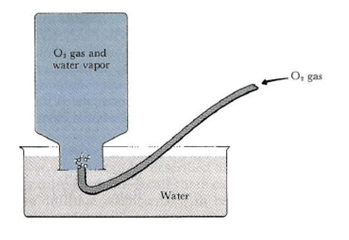## Collecting Gases Over Water

#### Learning Objective

• Apply Dalton’s Law to determine the partial pressure of a gas collected over water.

#### Key Points

• The total pressure in an inverted tube can be determined by the height of the water displaced in the tube.
• When calculating the amount of gas collected, Dalton’s Law must be used to account for the presence of water vapor in the collecting bottle.

#### Term

• pneumatic troughdevice used to collect a gas over water; the height of water displaced in the tube can be used to determine the total pressure inside the tube

Since gases have such small densities, it can be difficult to measure their mass. A common way to determine the amount of gas present is by collecting it over water and measuring the height of displaced water; this is accomplished by placing a tube into an inverted bottle, the opening of which is immersed in a larger container of water.Collecting gas over waterWhen collecting oxygen gas and calculating its partial pressure by displacing water from an inverted bottle, the presence of water vapor in the collecting bottle must be accounted for; this is easily accomplished using Dalton’s Law of Partial Pressures.

## The Pneumatic Trough

This arrangement is called a pneumatic trough, and it was widely used in the early days of chemistry. As the gas enters the bottle, it displaces the water and becomes trapped in the closed, upper part of the bottle. You can use this method to measure a pure gas (i.e. O2) or the amount of gas produced from a reaction. The collected gas is not the only gas in the bottle, however; keep in mind that liquid water itself is always in equilibrium with its vapor phase, so the space at the top of the bottle is actually a mixture of two gases: the gas being collected, and gaseous H2O. The partial pressure of H2O is known as the vapor pressure of water and is dependent on the temperature. To determine the quantity of gas we have collected alone, we must subtract the vapor pressure of water from the total vapor pressure of the mixture.

## Example 1

O2 gas is collected in a pneumatic trough with a volume of 0.155 L until the height of the water inside the trough is equal to the height of the water outside the trough. The atmospheric pressure is 754 torr, and the temperature is 295 K. How many moles of oxygen are present in the trough? (At 295 K, the vapor pressure of water is 19.8 torr.)

The total pressure in the tube can be written using Dalton’s Law of Partial Pressures:

${P}_{total}={P}_{{H}_{2}O}+{P}_{{O}_{2}}$

Rearranging this in terms of $P_{O_2}$, we have:

${P}_{{O}_{2}}= {P}_{total} - {P}_{{H}_{2}O}$

Because the height of the water inside the tube is equal to the height of the water outside the tube, the total pressure inside the tube must be equal to the atmospheric pressure. With substitution, we have:

$P_{O_2}=P_{total}-P_{H_2O}= 754 - 19.8 = 734\;torr = .966\;atm$

Next, we apply the Ideal Gas Law:

$n=\frac{PV}{RT}$

$=\frac {(.966 atm)(.155L)}{(.082 L\cdot atm\cdot {mol}^{-1}\cdot {K}^{-1}) (295K)}$

$=.00619 \; mol\;O_2$

## Example 2

Oxygen gas generated in an experiment is collected at 25°C in a bottle inverted in a trough of water. The external laboratory pressure is 1.000 atm. When the water level in the originally full bottle has fallen to the level in the trough, the volume of collected gas is 1750 ml. How many moles of oxygen gas have been collected?

If the water levels inside and outside the bottle are the same, then the total pressure inside the bottle equals 1.000 atm; at 25°C, the vapor pressure of water (or the pressure of water vapor in equilibrium with the liquid) is 23.8 mm Hg or 0.0313 atm.

Therefore, the partial pressure of oxygen gas is 1.000 – 0.031, or 0.969 atm.

The mole fraction of oxygen gas in the bottle is 0.969 (not 1.000), and the partial pressure of oxygen also is 0.969 atm. The number of moles is: $n=\frac{PV}{RT }=\frac{(.969 \ atm)( 1730\ cm^{3})}{(82.054\ cm^{3}\ K^{-1}\ mole^{-1}\ )( \ 298 \ K)}$

n = 0.068 moles O2